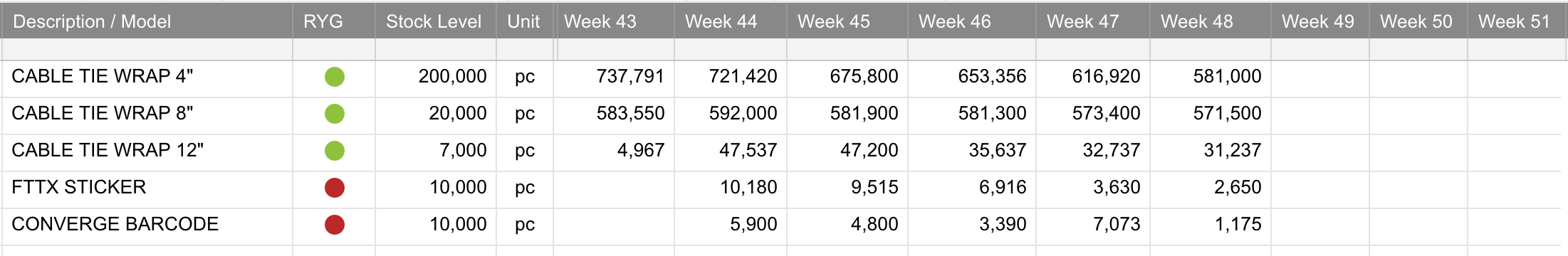# Auto Change Color or send a Notification/Mail

Hi Everyone,

As per the attached and formula, How can i add the formula if Week 43,43~52 below the stock Level, RYG ball become to red. Green above the stock level.

I'm only know the formula for single column.

=IF(ISBLANK([Stock Level]2), "Yellow", IF([Stock Level]2 <= [Week 43]2, "Green", IF([Stock Level]2 > [Week 43]2, "Red", "")))• Hello,

To include all of the columns in the same formula you could utilize an OR Function. https://help.smartsheet.com/function/or

You'll notice I replaced the row#'s with @row values, this helps prevent formula error when copying the formula from row to row. https://help.smartsheet.com/articles/2476491-create-efficient-formulas-with-at-cell

This could look like:

=IF(ISBLANK([Stock Level]@row), "Yellow", IF(OR([Stock Level]@row <= [Week 43]@row, [Stock Level]@row <= [Week 44]@row, [Stock Level]@row <= [Week 45]@row, [Stock Level]@row <= [Week 46]@row, [Stock Level]@row <= [Week 47]@row, [Stock Level]@row <= [Week 48]@row. [Stock Level]@row <= [Week 49]@row, [Stock Level]@row <= [Week 50]@row, [Stock Level]@row <= [Week 51]@row, [Stock Level]@row <= [Week 52]@row),"Green", IF(OR([Stock Level]@row > [Week 43]@row, [Stock Level]@row > [Week 44]@row, [Stock Level]@row > [Week 45]@row, [Stock Level]@row > [Week 46]@row, [Stock Level]@row > [Week 47]@row, [Stock Level]@row > [Week 48]@row, [Stock Level]@row > [Week 49]@row, [Stock Level]@row > [Week 50]@row, [Stock Level]@row > [Week 51]@row, [Stock Level]@row > [Week 52]@row),"Red", "")))

▸ BE AWARE !!!: If any of the values apply they will take precedence in regards to the order of operations. Which may not be the desired result.

For example, if 'Week 46' is "Green" but 'Week 47' is "Red" it will display as "Green" because 'Week 46' comes first in the formula. Currently, we don’t have a method to recognize each individual result and apply that result to the same symbol utilizing a formula.

To achieve the desired goal of having the Symbol column represent the color value of each 'Week'. You may want to create a Symbol column for each 'Week' utilizing the Original formula.

=IF(ISBLANK([Stock Level]@row), "Yellow", IF([Stock Level]@row <= [Week 43]@row, "Green", IF([Stock Level]@row > [Week 43]@row, "Red", "")))

Cheers,

Eric

Smartsheet Support